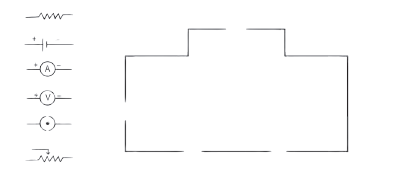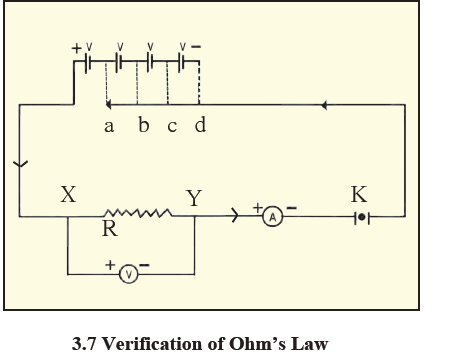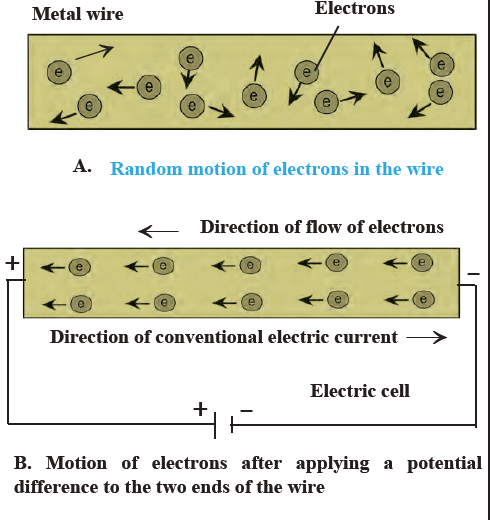# MSBSHSE Class 9 Science Chapter 3 Current Electricity Solutions

MSBSHSE Class 9 Science Chapter 3 Current Electricity Solutions, an important reference material from the viewpoint of your Class 9 Science examination of MSBSHSE, helps students to score well. The solutions, with detailed answers and step by step explanations provided here, will help students to understand the basic concepts of the chapter.

Current Electricity, an important concept of Science is discussed in detail in this chapter. The topics covered include Potential and potential difference, Electrical resistance and Ohm’s Law, Connection of resistors and effective resistance and more. Students can learn the subject thoroughly by referring to these MSBSHSE Class 9 Solutions of Science Chapter 3 Current Electricity. These solutions are prepared after proper research and will help students to get a thorough conceptual understanding. The well structured content makes it easier for the students to master the subject. This solution covers the topics as per the updated Maharashtra State Board Syllabus for Class 9. The solutions to the questions listed here are designed to help students ace the exams. Hence, practising these questions and giving highly relevant answers help the students to perform well.

## Maharashtra Board Class 9 Science Chapter 3- BYJU’S Important Questions & Answers

1.The following figure shows the symbol for components used in the accompanying electrical circuit. Place them at proper places and complete the circuit.Which law can you prove with the help of the above circuit?As given in the figure above, a continuous path of an electric current through conducting wires

is connected to the two ends of a cell and other resistances. This figure depicts how different

components are to be connected in the circuit, by using special symbols for each of the components. Such a figure is called an electric circuit diagram. This circuit helps to prove Ohm’s Law.

2. The following table shows current in Amperes and potential difference in Volts. Find the average resistance.

 V (Volts) I (Amp) 4 9 5 11.25 6 13.5

Answer: Given that Potential Difference V1 = 4 V and Current I1 = 9 A,

Then, calculate Resistance(R₁) = ?

Resistance(R₁)= V1/ I1

Hence, R₁= 4/9 = 0.44 Ω

Similarly, suppose V2 = 5 V and I2 = 11.25 A,

Then, Resistance(R₂) = V2/ I2

Thus, R₂ = 5/11.25 = 0.44 Ω

Also, assume V3 = 6 V and I3 = 13.5 A,

Resistance(R₃) = V3/ I3

Therefore,R₃= 6/13.5 = 0.44 Ω

Now, Find the Average Resistance (R) = (R₁ + R₂ + R₃)/3

R = (0.44 + 0.44 + 0.44)/3 = 0.44 Ω

Hence, the Average Resistance is 0.44 Ω.

3. Match the pairs

 ‘A’ GROUP ‘B’GROUP Free electrons V/ R Current Increases the resistance in the circuit Resistivity Weakly attached Resistances in series VA/LI

 ‘A’ GROUP ‘B’GROUP Free electrons Weakly attached Current V/ R Resistivity VA/LI Resistances in series Increases the resistance in the circuit

4. The resistance of a 1m long nichrome wire is 6 W. If we reduce the length of the wire to 70 cm. What will its resistance be?

Answer: Suppose resistance of nichrome =6 ohm and length=100 cm.

And given that R ∝ l

Now, 1m = 100 cm = 6 Ω, Hence, 70 cm= ?

100/ 70 = 60 / x

100 x = 70 × 6

Hence, X = (70 × 6) / 100 = 42/ 10

Thus, x=4.2 Ω

5. What is the use of electricity?

Answer: Electricity is used for operating electric furnaces, electric motors and several other instruments used in industries. Meanwhile, some of the other domestic appliances that function on electricity include fridge, electric oven, mixer, fans, washing machines, vacuum cleaner, roti maker and so on. These appliances have helped us by saving time and labour.

6. How do electrons flow?

Answer: Electrons flow from the point of lower potential to a point of higher potential.

7. What is Potential difference of a cell?

Answer: Potential difference of the cell is the difference in potential between the positive and negative terminals of a cell, caused by chemical reactions within the cell. The potential difference sets the electrons in motion and causes the flow of electricity using a conducting wire joined at the two ends of the cell. The amount of work done to carry a unit positive charge from point A to point B is known as the electric potential difference between the two points.

Therefore, Potential difference between two points = work / total charge transferred, V= W/ Q.

1V = 1J / 1C. Volt is the unit of potential difference in the SI system.

8. Who constructed the first electric cell?

Answer: Allesandro Volta, the Italian Scientists constructed the first electric cell. The unit of potential difference was termed volt in honour of the scientist.

9. What are free electrons?

Answer: Free electrons are the one or more outer shell electrons found in every atom of a metallic conductor, which are bound weakly to the nucleus. These electrons move smoothly from one part of a conductor to its other parts, also transferring its negative charge, as a result of the motion. The free electrons in a conductor are the carriers of negative charge.

10. Draw a diagram depicting the motion of free electrons.11. What is an electric current?

Answer: The flow of electrons through a conductor is termed an electric current. Current (I) is defined quantitatively as the charge passing through a conductor in unit time. Now, given that charge Q is flowing through the cross section in time (t), then Current (I) = Q / t . Columb (C) is the unit of charge in the SI unit. Meanwhile, current is expressed in Ampere.

12. A current of 0.4 A flows through a conductor for 5 minutes. How much charge would have passed through the conductor?

Answer: Here, I = 0.4 A, while t = 5 min = 5 × 60 s =300 s.

Now, apply the values to the formula Q = I × t. Hence,

Q= 0.4A × 300s =120C .

Thus, the charge passing through the conductor is 120C.

13. What is the physical state of a conductor?

Answer: Physical state of a conductor is its length, area of cross section, temperature and the material that it is made up of.

14. Explain Ohm’s law with the formula.

Answer: George Simon Ohm, a German Scientist put across a law known as Ohm’s law, from which the relationship between the current flowing through a wire (I) and the potential difference across its ends (V) can be obtained. As per the law, if the physical state of a conductor remains constant, the current (I) flowing through it is directly proportional to the potential difference (V) between its two ends. Given is the formula,

I ∝ V

I = kW ( k is the constant of proportionality)

Meanwhile, V = I × (1/k ) ( Here, 1/k = R =Resistance of the conductor)

So replace 1/k with R and you get

V= I × R = IR.

Thus, R = V/ I. This is ohm’s law.

15. What is Resistance of one Ohm?

Answer: The resistance of the conductor is one Ohm, if one Ampere current flows through a conductor when one Volt potential difference is applied between its ends.

16. What is resistance of a conductor?

Answer: A conductor contains a large number of free electrons that are constantly in random motion. Hence, when a potential difference is applied between two ends of the conductor, these electrons start moving from the end at lower potential to the other end at higher potential, causing the flow of current. These moving electrons strike the atoms and ions that lie along their path resulting in hindrance to the flow of electrons and thus, opposing the current. This hindrance is called the resistance of the conductor.

17. Mention the resistivity of Copper, Nichrome and Diamond.

Answer: Resistivity of copper is given as 1 7 × 10-8 Ω m, while that of nichrome is 11 × 10-6 Ω m and for diamond is 162 × 10-13 Ω m to 162 × 10-18 Ω m.

18. What is the use of an Open tap key or plug key of an electric circuit?

Answer: Open tap key or plug key of an electric circuit is used to curb the flow of current flowing in a circuit by disconnecting two ends of a wire.

19. What are conductors and Insulators?

Answer: Substances can be divided into conductors and insulators based on their resistance to electric current. The substances that have very low resistance are called conductors and current can easily flow through these materials. Meanwhile, Insulators have extremely high resistance and current cannot flow through them.

20. What are Ammeter and Voltmeter?

Answer: Ammeter is used to measure the current flowing in the circuit, while Voltmeter can be used to measure the potential difference between two points in the circuit.

21. The resistance of the filament in a light bulb is 1000 Ω. If the bulb is fed by a current from a source of potential difference 230 V, how much current will flow through it?

Answer: Here, the R is given as 1000 Ω, while V = 230 V. Hence, applying the formula I = V/R and replacing the values given, I = 230 v / 1000 Ω = 23/ 100= 0.23 A

22. The length of a conducting wire is 50 cm and its radius is 0.5 mm. If its resistance is 30 Ω, what is the resistivity of its material?

Answer: Given that, L = 50 cm = 50 × 10-2 m, r = 0.5 mm = 0.5 × 10-3,m = 5 × 10-4,m and R= 30 Ω, apply the formula for resistivity ρ = RA / L and A = π r2. Hence,

ρ = R × π r2/ L = 30 × 3.14 × ( 5 × 10-4)2,/ 50 × 10-2

= 30 × 3.14 × 25 × 10-8,/ 50 × 10-2

= 3 × 3.14 × 25 × 10-9/ 5 × 10-3

= 3 × 3.14 × 25 × 10-6/ 5 = 47.1 × 10-6 Ω m

= 4.71 × 10-5 Ω m

Thus, resistivity of the wire is 4.71 × 10-5 Ω m

23. A current of 0.24 A flows through a conductor when a potential difference of 24 V is applied between its two ends. What is its resistance?

Answer: Suppose V= 24 V and I = 0.24 A. Given that R = V / I is the formula to apply, replace the values specified.

R = V/ I = 24/ 0.24

Hence, R = 100 Ω. Thus, the resistance of the conductor is 100 Ω.

24. Determine the current that will flow when a potential difference of 33 V is applied between two ends of an appliance having a resistance of 110 Ω. If the same current is to flow through an appliance having a resistance of 500 Ω, how much potential difference should be applied across its two ends?

Answer: If V= 33 V and R= 110 Ω, then in the first case, formula used is

I = V/ R = 33 /110 = 0.3 A.

Hence, the current flowing through the appliance is 0.3 A.

Similarly, In the second part, you are required to determine the potential difference that should be applied across its two ends, so the formula to use V = IR . Here, I= 0.3 A and R is already given as 500 Ω.

Therefore, V = IR = 0.3 × 500 V= 150V.

Hence, the required potential difference between its two ends is 150V.

25. Determine the resistance of a copper wire having a length of 1 km and

diameter of 0.5 mm.

Answer: Here, Resistivity of copper is given as ρ= 1.7 × 10-8 Ω m. (Convert all the given measures into meters). L = 1 km = 1000 m = 103 m

d = 0.5 mm = 0.5 × 10-3 m

Now, if r is given as the radius of the wire, then the area of its cross section is given by formula A = π r2

Hence, A = π (d/2) 2

A = π /4 ( 0.5 × 10-3) 2,m2

= 0.2 × 10-6

So, replace the values to the formula

ρ = RA / L = (1.7 × 10-8 Ω m × 10-3m) / 0.2 × 10-6m2,= 85 Ω.

26. What is a resistor?

Answer: A two ended component having a given amount of resistance between its

two ends are known as a resistor.

27. Three resistors having resistances of 15 Ω, 3 Ω and 4 Ω are connected in

series. What is the effective resistance in the circuit?

Answer: Here, R1= 15 Ω, R2= 3 Ω and R3=4 Ω are given. Now, for the effective resistance of these resistors connected in series R = R1+ R2+ R3.

Hence, R = 15 + 3 + 4 = 22 Ω.

Thus, the effective resistance in the circuit is 22 Ω.

28. Two resistors having resistances of 16 Ω and 14Ω are connected in series.

If a potential difference of 18 V is applied across them, calculate the current flowing through the circuit and the potential difference across each individual resistor.

Answer: Here, R1 = 16 Ω and R2=14Ω ,

So, effective Resistance RS = R1+ R2 = 16 + 14 = 30 Ω.

Now, the current flowing through the circuit is I, while the potential difference across 16 Ω and 14Ω will be V1 and V2, respectively. Considering the formula

V = IRs ( Given that V = V1+V2= 18V)

I = V/RS = 18 V/ 30 Ω

Thus, I= 0.6 A

Now, V1= I×R1

V1= 0.6 × 16= 9.6V

Similarly, V2= I ×R2= 0.6 × 14= 8.4 V

Therefore, the current flowing through the circuit is 0.6A and the potential differences across the resistances 16 Ω and 14Ω are 9.6V and 8.4 V, respectively.

29. Explain super conductors and non-ohmic conductors.

Answer: The conductors whose resistance becomes nearly zero if their temperature is decreased upto a certain value close to 0 K are called superconductors. Meanwhile, there are other conductors that do not obey Ohm’s law. They are known as non-ohmic conductors.

30. Resistors having resistances of 15 Ω, 20 Ω and 10 Ω are connected in

parallel. What is the effective resistance in the circuit?

Answer: Given that R1= 15 Ω, R2= 20 Ω and R3= 10 Ω ,

Now to get the effective resistance of the resistors connected in parallel,

I /RP= 1/ R1 + 1/R2 + 1/R3

Hence, I /RP = 1/ 15 + 1/20 + 1/10 = (4+3+6) / 60 = 13/ 60

RP, = 13/60 = 4.615 Ω

Thus, the effective resistance in the circuit = 4.615 Ω, which is less than the least of the three (10Ω)

31. The electricity in our homes is brought through the main conducting cable either from the electric pole or from underground cables. Usually, there are three wires in the cable. Which are they?

Answer: One is called the live wire with the red or brown insulation and this brings in the current. Then, there is the blue or black neutral wire through which the current returns. In India, the difference between the live and neutral wires is nearly 220 V. Finally, the third wire is of yellow or green colour and is called the earth wire.This is used for safety purposes and is connected to a metal plate buried deep underground near the house.

32. Why are fuse wires used?

Answer: Used to protect domestic appliances, fuse wire has specific melting point and is made of a mixture of substances. It is connected in series to the electric appliances. When the current in the circuit increases excessively, then the fuse wire is heated up and melts, breaking the circuit and stopping the flow of current, thus protecting the appliance.

33. What are the precautions to be taken while using electricity?

Answer: Here, are some precautions to take while using electricity:

1. Keep electric switches and sockets away from small children, fitting them at height where they cannot reach and put pins or nails inside. Do not pull the plug wires while removing a plug from its socket.

2. Switch of the electrical appliance before cleaning it and also remove its plug

from the socket.

3. Do not handle electrical appliances with wet hands and remember to use footwear with rubber soles. Rubber is an insulator and it can help to prevent the current from flowing through our body, thereby protecting it.

4. Finally, avoid touching the person with electric shock. Put off the main switch and in case the switch is too far or you have no idea where it is located, then remove the plug from the socket, if possible. If you are not able to then use a wooden pole and push the person away from the electric wire.

Meanwhile, students who are studying in Class 9 should know the syllabus thoroughly so that they can prepare well and excel in their exam. Keep visiting BYJU’S to get the detailed Maharashtra Board syllabus, Maharashtra state Board books, Question papers, Sample papers, etc.

## Frequently Asked Questions on Maharashtra State Board Solutions for Class 9  Science Chapter 3 Current Electricity

### How useful are these Maharashtra Board Class 9 Science Chapter Solutions?

These solutions are very useful. Students are encouraged to practise these questions at first. Then they can refer back to the solutions to analyse their performance. This will also help them to rectify the mistakes, so that they can avoid making any during the board exams. These solutions also set the basis for some of the questions that could get asked in the board exams for Science paper.